# Where and when

The truck left Kremnica at 11:00 h at a speed of 60km/h. At 12:30 h, the passenger car started at an average speed of 80km/h. How many kilometers from Kremnica will the passenger car reach the truck, and when?

Result

s =  360 km
T = 17:00 hh:mm### Step-by-step explanation: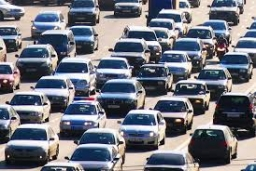Did you find an error or inaccuracy? Feel free to write us. Thank you!Tips to related online calculators
Do you have a linear equation or system of equations and looking for its solution? Or do you have a quadratic equation?
Do you want to convert velocity (speed) units?
Do you want to convert time units like minutes to seconds?

## Related math problems and questions:

• Passenger car and truckFrom Kutna Hora left at 11:00 clock a truck at 60 km/h. At 12:30 behind him started passenger car at average speed 80 km/h. At what time and how far from Kutna Hora overtake a passenger car truck?
• Motorcyclist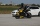From Trutnov, the motorcyclist started at an average speed of 60km/hour. At 12.30hrs the passenger car was started at a speed of 80km/hour. How many hours and at what distance from Trutnov will car catch a motorcycle?
• MotionFrom two different locations distant 232 km started against car and bus. The car started at 5:20 with average speed 64 km/h. Bus started at 7:30 with average speed 80 km/h. When they meet? How many kilometers went the bus?
• Truck and car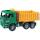At 6h45min, the truck started at a speed of 36 km/h. At 7h30m behind it a passenger car. Find the passenger car's average speed to catch the truck 72 km away from the factory.
• Škoda carsAt 8:30 am, Škoda 120 started from Prievidza at 60 km/h in the direction of Bratislava. At 9:00 started from Prievidza at the same direction Škoda Rapid at 90 km/h. Where and when will Škoda Rapid catch up Skoda 120?
• Motion 2At 10 o'clock started from place A car speed at 90 km/h. Twenty minutes after starting another car from place B at a speed 60 km/h . Distance places A and B is 120 kilometers. What time and where do they meet?
• The tourist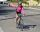The tourist came started from the hostel at an average speed of 5km/h. Half an hour later, the bicyclist started along the same route at a speed of 20km/h. How many minutes will a cyclist catch up and how many kilometers will he go?
• Brno-Tachov highwayThe distance Brno-Tachov is 360 km. At 8:00 a. M. , a car travels from Brno on the highway, traveling at an average speed of 90 km/h. An hour later, a car leaves Tachov towards Brno, whose average speed is 110 km/h. Calculate how far apart they will be at
• Truck PRG-BA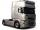Truck driving on the highway from Prague to Bratislava at an average speed of 72 km/h. At the moment when the truck passes 54 kilometers from Prague passenger car departs from Prague, which travels to Bratislava at a speed of 90 km/h. When and where (at w
• Cars motion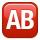From point A car start at the speed of 90 km/h. Against it departs from the point B at the same time another car at 60 km/h. For how long and where they met if the distance between A and B is 180 km?
• Two citiesThe distance between cities A and B is 132 km. At 9.00 am, the cyclist started the bike at an average speed of 24 km/h, and at 10.00 am started from the B cyclist at an average speed of 30 km/h. How long and far from A will they both meet?
• Average speed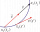The car drove on one section of the highway for half an hour at a speed of 80 km/h. Then he reduced his speed to 60km/h. It went three-quarters of an hour at a speed of 60 km/h. What was the average speed of the car?
• Two cars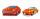From the town A to town B started two cars. The first at 7:00 at average speed 60 km per hour, the second at 10:00 at average speed 100 km per hour. The first car will not stay in B, and on the way back meet the second car at half way from A to B. At what
• Cars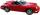Cars started at 7:00 AM. From point A car run at 40 km per hour, from B car run 70 km per hour. Between A and B is a 250 km long road. When and where will meet (measured from point A)?
• Two trains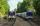Two trains go at each other. First at speed 40 km/h and the second at speed 60 km/h. At what distance will be 30 minutes before they will meet?
• Tourists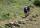From the cottage started first group of tourists at 7:00 AM at speed 4 km/h. The second started after them 21 minutes later at speed 6 km/h. How long and how many kilometers from the cottage will catch the first group?
• Two citiesCities A and B are 200 km away. At 7 o'clock from city A, the car started at an average speed of 80 km/h, and from B at 45 min later, the motorcycle is started at an average speed of 120 km/h. How long will they meet, and at what distance from point A wil